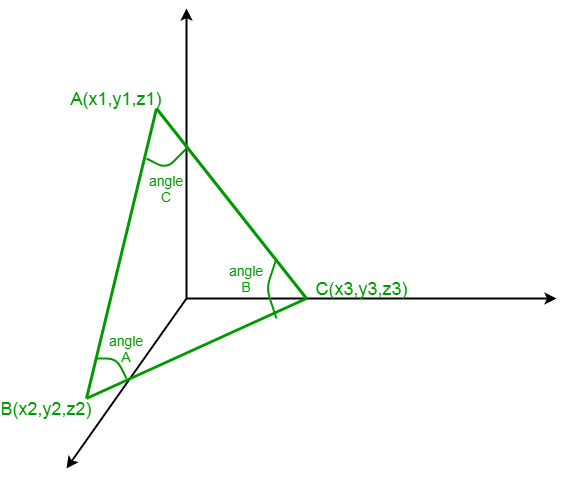# Find all angles of a triangle in 3D

Given coordinates of 3 vertices of a triangle in 3D i.e. A(x1, y1, z1), B(x2, y2, z2), C(x3, y3, z3). The task is to find out all the angles of the triangle formed by above coordinates.

Examples:

Input:
x1 = -1, y1 = 3, z1 = 2
x2 = 2, y2 = 3, z2 = 5
x3 = 3, y3 = 5, z3 = -2

Output:
angle A =  90.0 degree
angle B =  54.736 degree
angle C =  35.264 degree## Recommended: Please try your approach on {IDE} first, before moving on to the solution.

Approach:
For finding angle A find out direction ratios of AB and AC :
direction ratios of AB = x2-x1, y2-y1, z2-z1
direction ratios of AC = x3-x1, y3-y1, z3-z1
then angle A =For finding angle B find out direction ratios of BA and BC :
direction ratios of BA = x1-x2, y1-y2, z1-z2
direction ratios of BC = x3-x2, y3-y2, z3-z2
then angle B =For finding angle C find out direction ratios of CB and CA :
direction ratios of CB = x2-x3, y2-y3, z2-z3
direction ratios of CA = x1-x3, y1-y3, z1-z3
then angle C =Below is the implementation of above approach:

## C++

 //CPP program for finding all angles of a triangle   #include  #include  using namespace std;     // function for finding the angle   float angle_triangle(int x1, int x2, int x3,               int y1, int y2, int y3, int z1, int z2, int z3)  {         int num = (x2-x1)*(x3-x1)+(y2-y1)*(y3-y1)+(z2-z1)*(z3-z1) ;         float den = sqrt(pow((x2-x1),2)+pow((y2-y1),2)+pow((z2-z1),2))*\                  sqrt(pow((x3-x1),2)+pow((y3-y1),2)+pow((z3-z1),2)) ;         float angle = acos(num / den)*(180.0/3.141592653589793238463) ;         return angle ;  }     // Driver code  int main()  {  int x1 = -1;  int y1 = 3;  int z1 = 2;  int x2 = 2;  int y2 = 3;  int z2 = 5;  int x3 = 3;  int y3 = 5;  int z3 = -2;  float angle_A = angle_triangle(x1, x2, x3, y1, y2,                                  y3, z1, z2, z3);  float angle_B = angle_triangle(x2, x3, x1, y2, y3,                                   y1, z2, z3, z1);  float angle_C = angle_triangle(x3, x2, x1, y3,                               y2, y1, z3, z2, z1);  cout<<"Angles are :"<

## Java

 //Java program for finding all angles of a triangle      class GFG{  // function for finding the angle   static double angle_triangle(int x1, int x2, int x3,               int y1, int y2, int y3, int z1, int z2, int z3)  {         int num = (x2-x1)*(x3-x1)+(y2-y1)*(y3-y1)+(z2-z1)*(z3-z1) ;         double den = Math.sqrt(Math.pow((x2-x1),2)+                  Math.pow((y2-y1),2)+Math.pow((z2-z1),2))*                  Math.sqrt(Math.pow((x3-x1),2)+                  Math.pow((y3-y1),2)+Math.pow((z3-z1),2)) ;         double angle = Math.acos(num / den)*(180.0/3.141592653589793238463) ;         return angle ;  }     // Driver code  public static void main(String[] args)  {  int x1 = -1;  int y1 = 3;  int z1 = 2;  int x2 = 2;  int y2 = 3;  int z2 = 5;  int x3 = 3;  int y3 = 5;  int z3 = -2;  double angle_A = angle_triangle(x1, x2, x3, y1,                              y2, y3, z1, z2, z3);  double angle_B = angle_triangle(x2, x3, x1,                              y2, y3, y1, z2, z3, z1);  double angle_C = angle_triangle(x3, x2, x1,                              y3, y2, y1, z3, z2, z1);  System.out.println("Angles are :");   System.out.println("angle A = "+angle_A+" degree");  System.out.println("angle B = "+angle_B+" degree");  System.out.println("angle C = "+angle_C+" degree");  }  }  // This code is contributed by mits 

## Python3

 # Python Code for finding all angles of a triangle   import math      # function for finding the angle   def angle_triangle(x1, x2, x3, y1, y2, y3, z1, z2, z3):          num = (x2-x1)*(x3-x1)+(y2-y1)*(y3-y1)+(z2-z1)*(z3-z1)          den = math.sqrt((x2-x1)**2+(y2-y1)**2+(z2-z1)**2)*\                  math.sqrt((x3-x1)**2+(y3-y1)**2+(z3-z1)**2)          angle = math.degrees(math.acos(num / den))          return round(angle, 3)      # driver code       x1 = -1 y1 = 3 z1 = 2 x2 = 2 y2 = 3 z2 = 5 x3 = 3 y3 = 5 z3 = -2 angle_A = angle_triangle(x1, x2, x3, y1, y2, y3, z1, z2, z3)   angle_B = angle_triangle(x2, x3, x1, y2, y3, y1, z2, z3, z1)   angle_C = angle_triangle(x3, x2, x1, y3, y2, y1, z3, z2, z1)   print("Angles are :")   print("angle A = ", angle_A, "degree")   print("angle B = ", angle_B, "degree")   print("angle C = ", angle_C, "degree")  

## C#

 // C# program for finding all   // angles of a triangle   using System;                         class GFG  {         // function for finding the angle   static double angle_triangle(int x1, int x2, int x3,                                int y1, int y2, int y3,                                int z1, int z2, int z3)  {         int num = (x2 - x1) * (x3 - x1) +                (y2 - y1) * (y3 - y1) +                 (z2 - z1) * (z3 - z1);         double den = Math.Sqrt(Math.Pow((x2 - x1), 2) +                             Math.Pow((y2 - y1), 2) +                              Math.Pow((z2 - z1), 2)) *                   Math.Sqrt(Math.Pow((x3 - x1), 2) +                              Math.Pow((y3 - y1), 2) +                              Math.Pow((z3 - z1), 2));         double angle = Math.Acos(num / den) *                      (180.0/3.141592653589793238463);         return angle ;  }     // Driver code  public static void Main()  {      int x1 = -1, y1 = 3, z1 = 2;      int x2 = 2, y2 = 3, z2 = 5;      int x3 = 3, y3 = 5, z3 = -2;      double angle_A = angle_triangle(x1, x2, x3,                                       y1, y2, y3,                                       z1, z2, z3);      double angle_B = angle_triangle(x2, x3, x1,                                      y2, y3, y1,                                       z2, z3, z1);      double angle_C = angle_triangle(x3, x2, x1,                                      y3, y2, y1,                                      z3, z2, z1);      Console.WriteLine("Angles are :");       Console.WriteLine("angle A = " + angle_A +                                      " degree");      Console.WriteLine("angle B = " + angle_B +                                     " degree");      Console.WriteLine("angle C = " + angle_C +                                      " degree");  }  }     // This code is contributed by 29AjayKumar 

## PHP

  

Output:

Angles are :
angle A =  90.0 degree
angle B =  54.736 degree
angle C =  35.264 degree
`

My Personal Notes arrow_drop_upCheck out this Author's contributed articles.

If you like GeeksforGeeks and would like to contribute, you can also write an article using contribute.geeksforgeeks.org or mail your article to contribute@geeksforgeeks.org. See your article appearing on the GeeksforGeeks main page and help other Geeks.

Please Improve this article if you find anything incorrect by clicking on the "Improve Article" button below.